# The Communication Complexity of Threshold Private Set Intersection-2019：解读

Alice和Bob当两者的交集大小不小于$$n-t$$时（两者的不同元素的数量不大于阈值$$2t$$时），才会求交集。

1、检测
2、求交

## 摘要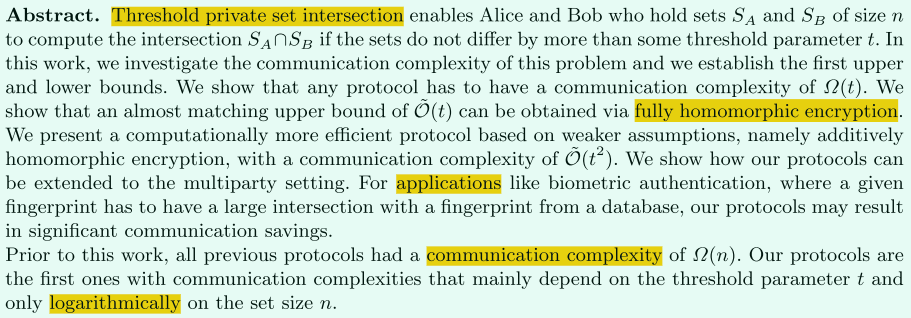1、简单介绍了阈值PSI
2、分析了阈值PSI协议的通信复杂度的上下界。
3、两方协议可以扩展到多方
4、阈值PSI应用广泛
5、给出协议的通信复杂度取决于阈值$$t$$，并和集合大小$$n$$呈亚线性关系（对数）

## 介绍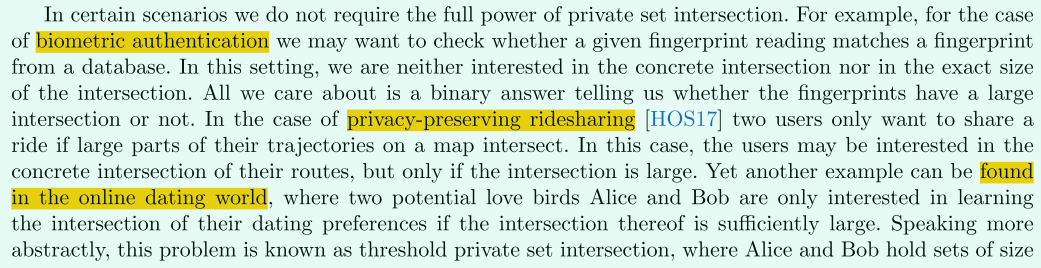1、阈值PSI的应用
（1）生物特征认证

（2）隐私拼车

（3）在线聊天好友发现

2、以往的PSI的通信复杂度分析
（1）通信复杂度与最小输入集合大小呈线性
（2）而阈值PSI通信复杂度只取决于阈值大小，而与输入集合大小无关。

3、主要技术
（1）Cardinality Testing
（2）Linear Algebra
（4）Secure Two-Party Computation
（5）Oblivious Linear Function Evaluation
（6）set reconciliation【Set reconciliation with nearly optimal communication complexity】

4、文章框架
（1）先介绍基础知识
（2）再分析文章的通信复杂度
（3）再基于FHE和set reconciliation构造的协议
（4）再介绍不安全的Cardinality Testing
（5）再介绍安全的Cardinality Testing
（6）再给出不安全的两方阈值PSI
（7）再给出安全的两方阈值PSI
（8）最后给出扩展多方阈值PSI的设想方案

## 基础知识

Alice将集合$$S_A=(a_1,...,a_n)$$编码为多项式$$p_A(x)=\prod_{i=1}^{n}(x-a_i)$$，Bob将集合$$S_B=(b_1,...,b_n)$$编码为多项式$$p_B(x)=\prod_{i=1}^{n}(x-b_i)$$

### 代数基础

1、多项式
（1）单项式（monomials）

（2）多项式级数（deg(p)）

（3）首一多项式（monic）

（4）多项式的稀疏性（t-sparse）

（5）有理多项式（rational polynomial或rational function）
$$h(x)=p(x)/q(x)$$就是有理多项式，其中$$p(x)$$$$q(x)$$的级数分别为n和m
（6）多项式插值

2、矩阵
（1）奇异矩阵（singular）

（2）汉克尔矩阵（Hankel matrix）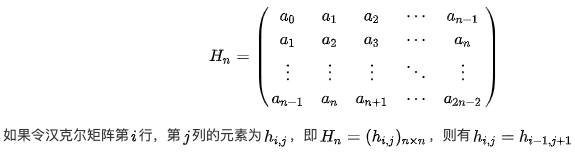### 引理

1、引理1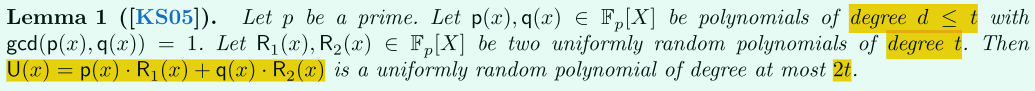2、引理2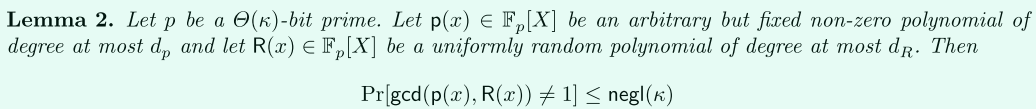### set reconciliation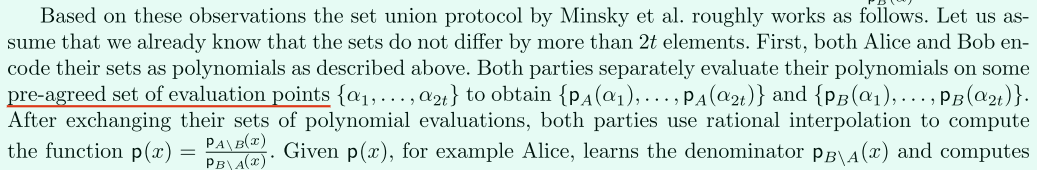（1）双方输入多项式的计算点
（2）使用安全两方计算对$$p(x)$$进行插值
（3）两方分别输出$$p_{A\setminus B}(x),p_{B\setminus A}(x)$$

#### 不安全的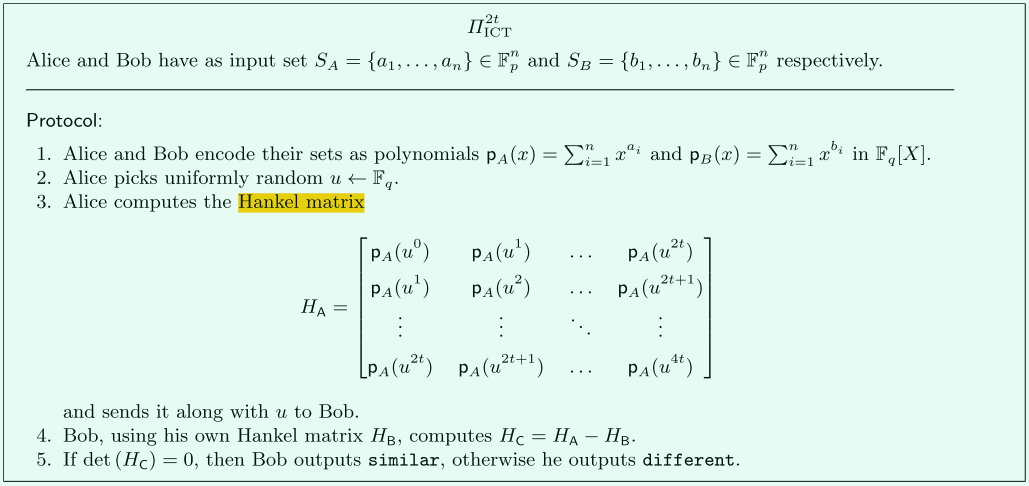#### 安全的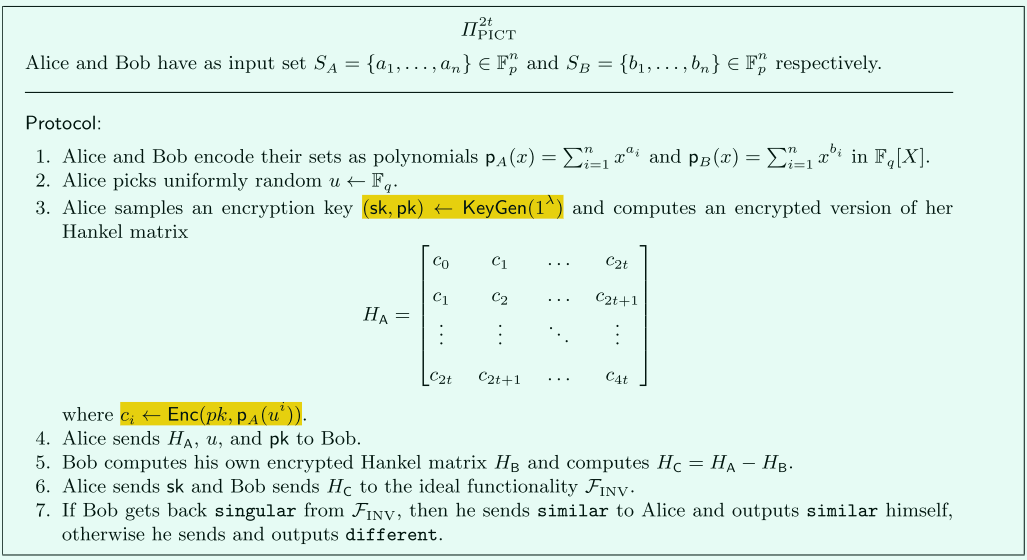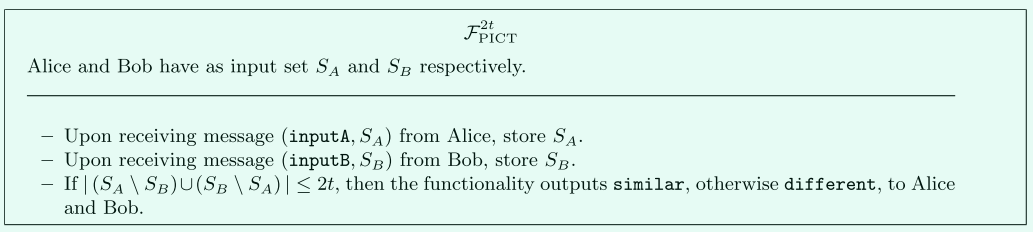### 协议通信量1、使用FHE构造的阈值PSI的通信复杂度最多为$$\widetilde{O}(t)$$，但在密文下进行插值多项式，计算量巨大。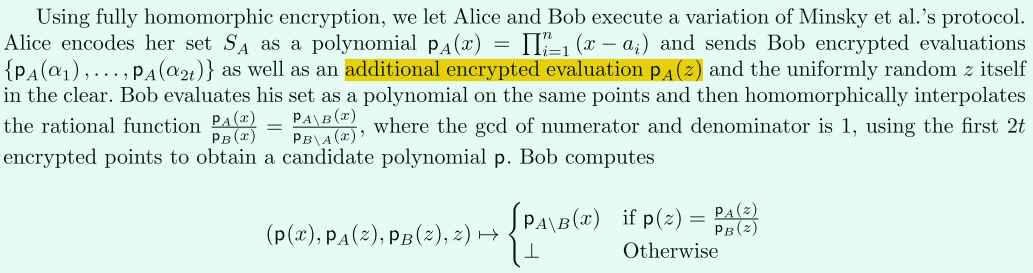2、使用GC构造的阈值PSI的通信协议为$$\widetilde{O}(t^3)$$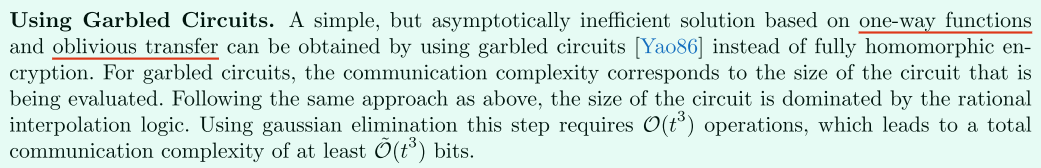3、我们的协议介于两者之间，比2更优，同时使用加法同态（部分同态）在保证计算复杂度可控内，实现高效通信。

## 主要内容

### 两方阈值PSI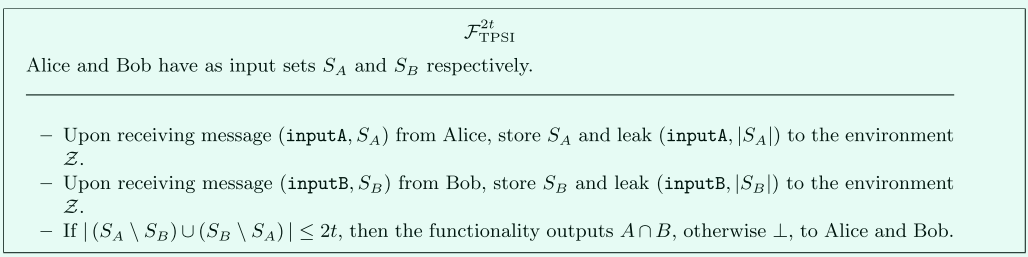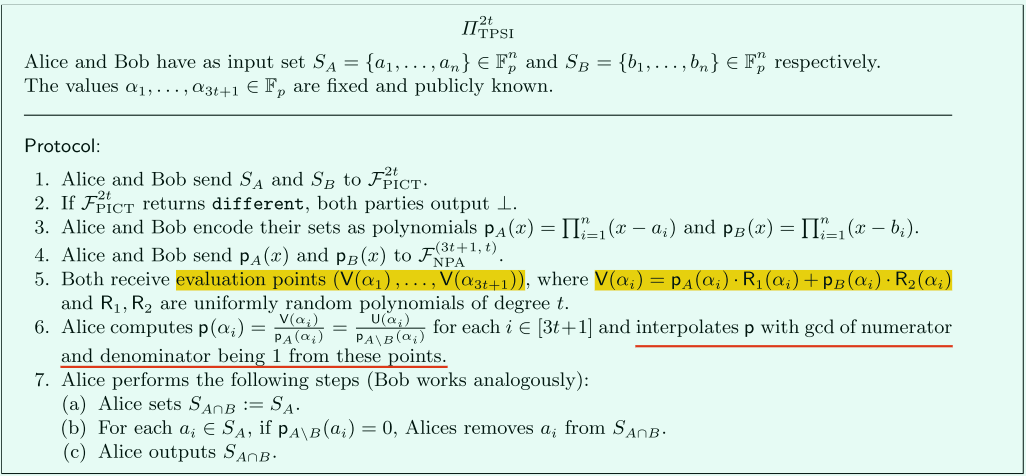#### 非对称场景下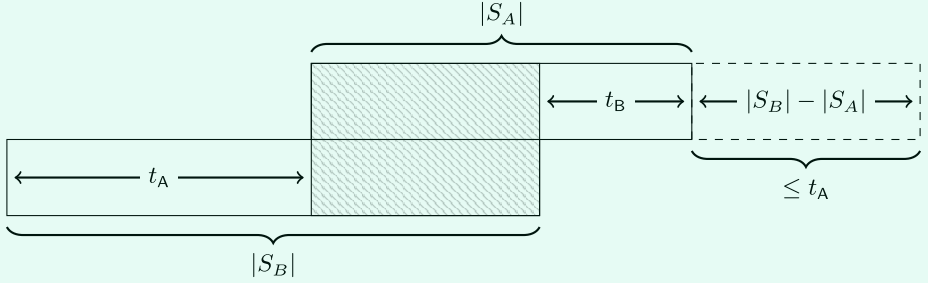#### 加入“噪音”1、输入
sender：编码得到多项式$$p_A(x)$$和随机多项式$$R(x)$$
receiver：编码得到多项式$$p_B(x)$$
2、输出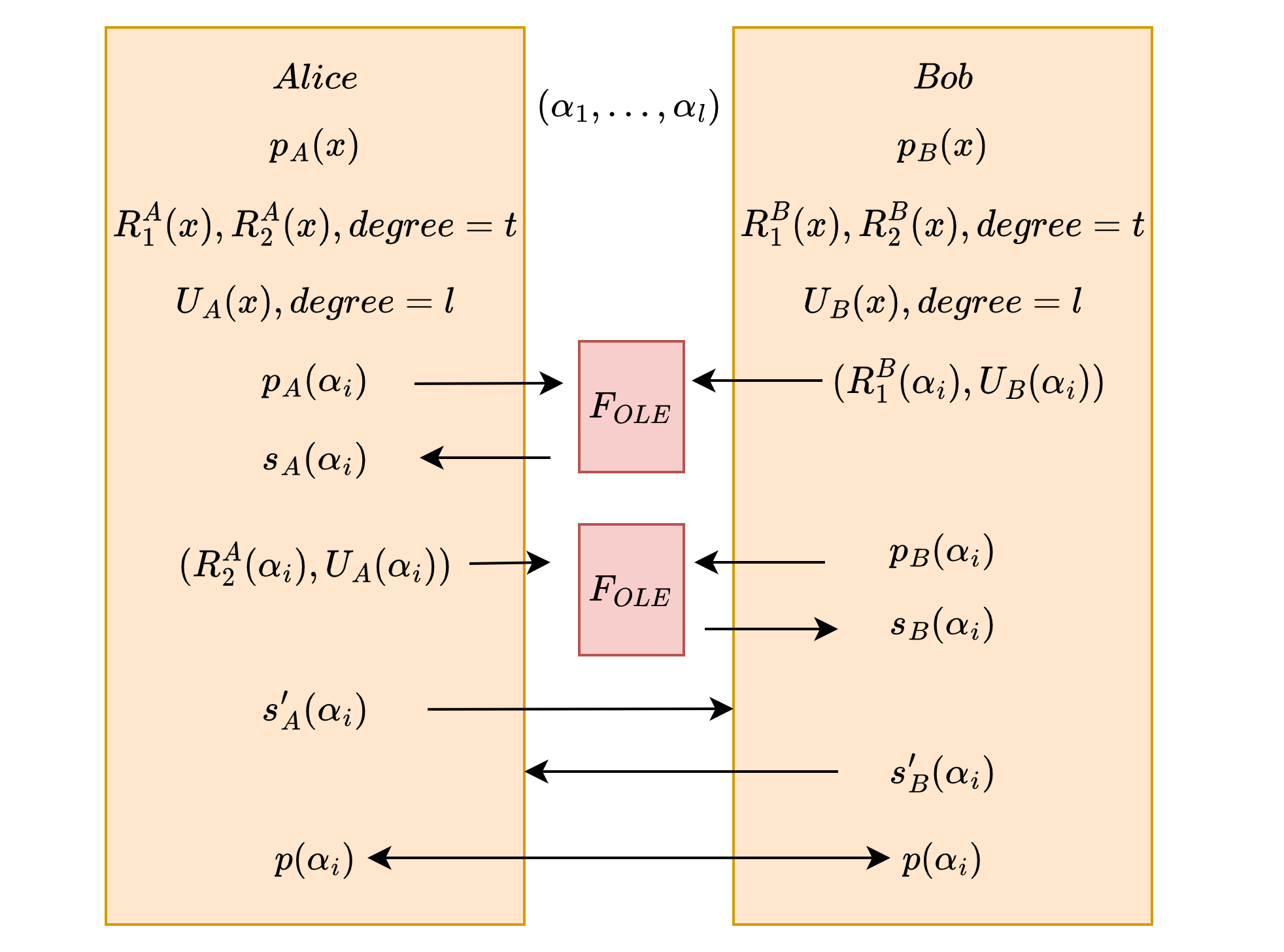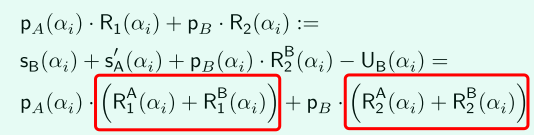### 多方阈值PSI

Alice，Bob，张三，数据集分别是$$S_A,S_B,S_C$$（大小为n），编码为多项式$$p_A(x)=\prod_{i=1}^{n}(x-a_i),p_B(x)=\prod_{i=1}^{n}(x-b_i),p_C(x)=\prod_{i=1}^{n}(x-c_i)$$

#### 检测（Cardinality Testing）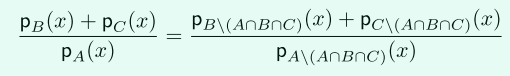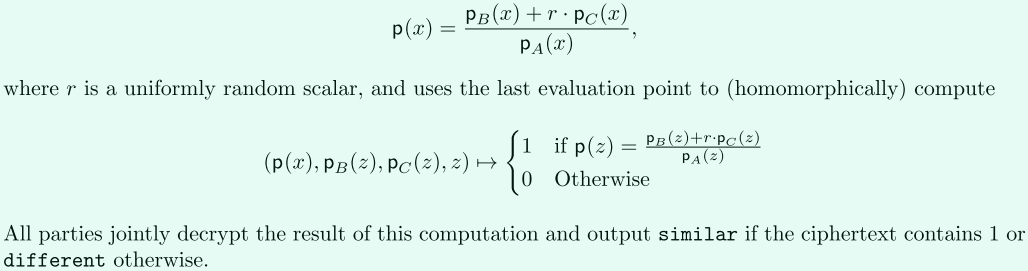1、Bob和张三分别发送加密的$$(p_B(\alpha_1),...p_B(\alpha_{2t}),p_B(z)),(p_C(\alpha_1),...p_C(\alpha_{2t}),p_C(z))$$给Alice，其中$$z$$是一个随机值。
2、Alice使用这$$2t$$个点值对进行同态插值，求出$$p(x)$$，并带入$$z$$，判断$$p(z)$$是否等于$$(p_B(z)+rp_C(z))/p_A(z)$$，如果相等则为1的加密，否则是0的加密。
3、最后三方联合解密，做最后判断。

#### 求交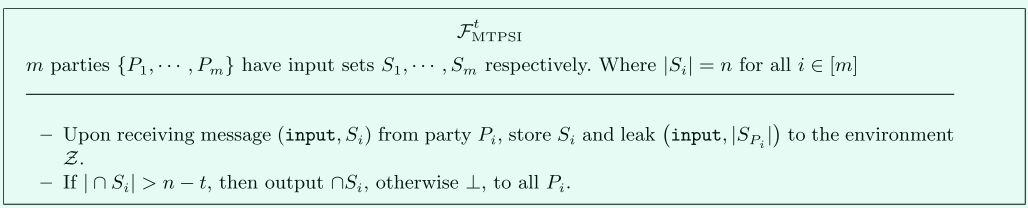1、Alice，Bob，张三输入自己的数据：$$(p_A(x),R_1^A(),R_2^A(),R_3^A(),U_A()),(p_B(x),R_1^B(),R_2^B(),R_3^B(),U_B()),(p_C(x),R_1^C(),R_2^C(),R_3^C(),U_C())$$
2、各自得到$$3t+1$$个点$$(\alpha_1,...,\alpha_{3t+1})$$值对$$p_A(x)R_1(x)+p_B(x)R_2(x)+p_C(X)R_3(x)$$
3、以Alice为例，Alice最后插值出$$p(x)$$，进而求出$$p_{A\setminus (A\cap B \cap C)}(x)$$，最后求出交集。## 总结

1、插值是在密文域，所以计算量很大
2、检测和求交中的同态加密方案，可以用同一个，比如TFHE
3、该论文只是给出一个模型、思路，具体实用性不强。
4、后续在下一篇论文介绍改进，增强实用性！

## 参考

1、什么是亚线性和超线性？
（1）线性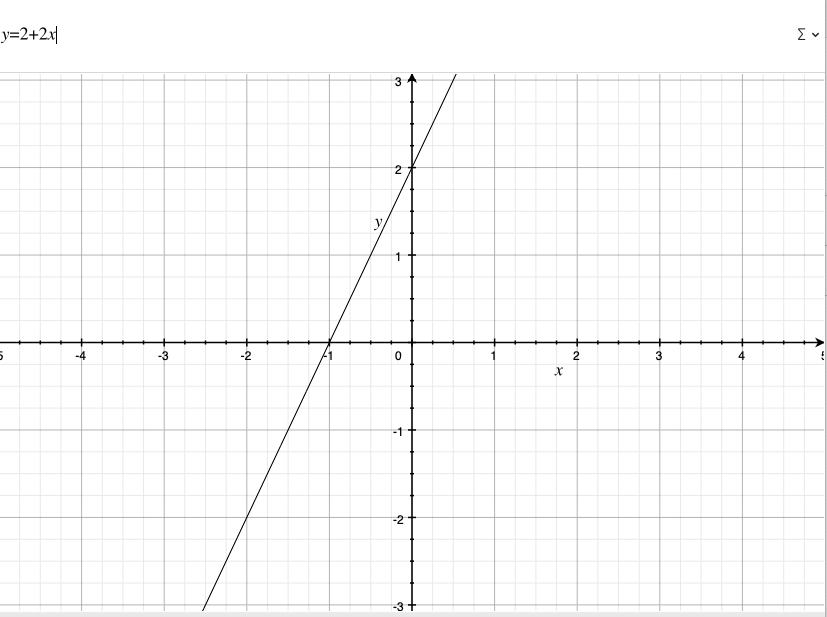（2）亚线性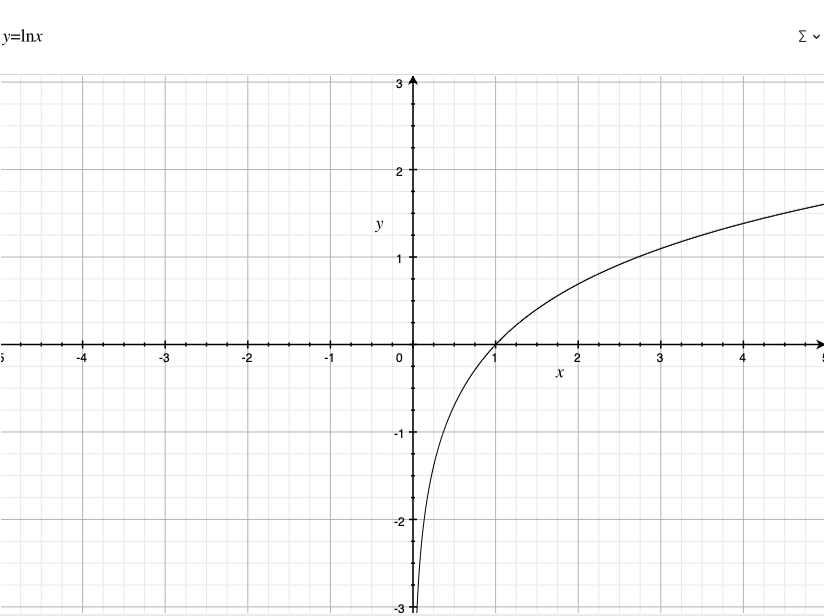（3）超线性2、数学当中的非平凡解和平凡解如何理解？非平凡性呢？
（1）平凡

（2）非平凡

3、渐进标准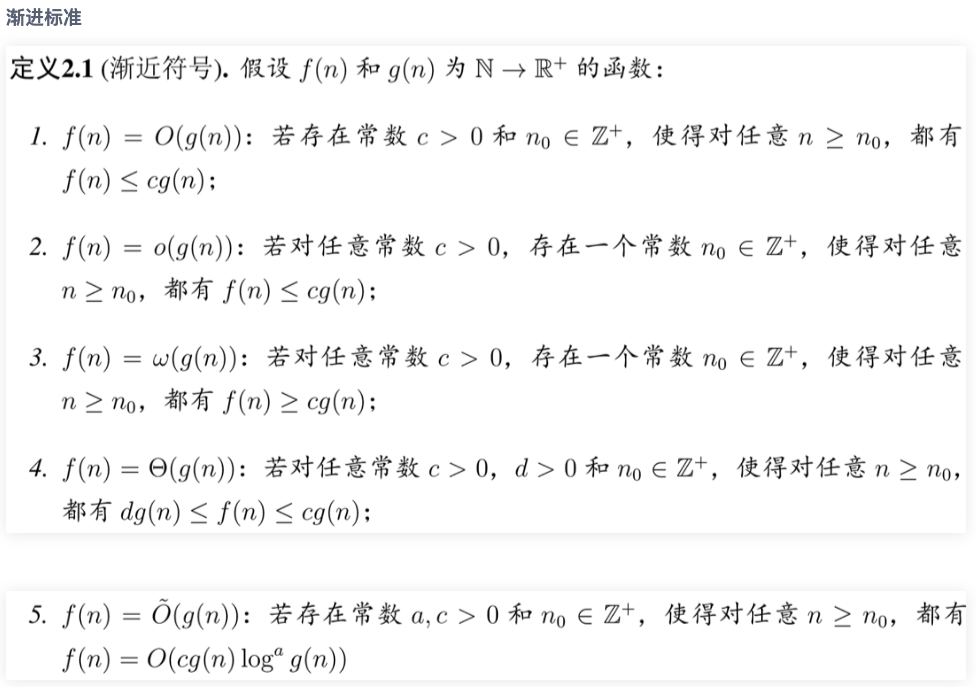posted @ 2022-06-23 15:48  PamShao  阅读(14)  评论(0编辑  收藏  举报